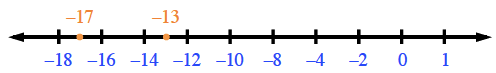### Home > CC1MN > Chapter 10 > Lesson 10.3.1 > Problem10-77

10-77.

Use $<, >,$or $=$ to compare the number pairs below.

1. $0.183 \text{ ____ } 0.18$

Write both numbers to the thousandths place, then compare.

1. $-13\text{ ____}-17$

It may help to place the numbers on a number line, like the one below.1. $0.125 \text{____}\frac { 1 } { 8 }$

Convert the fraction to a decimal, then compare.

1. $-6\text{ ____}-4$

See part (b).

1. $72\%\text{ ____ }\frac { 35 } { 30 }$

Examine the fraction; is it greater than or less than 1?

1. $-0.25\text{ ____}-0.05$

See part (b).